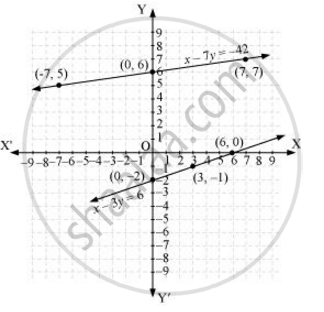# Aftab tells his daughter, “Seven years ago, I was seven times as old as you were then. Also, three years from now, I shall be three times as old as you will be.” (Isn’t this interesting?) Represent this situation algebraically and graphically - Mathematics

Aftab tells his daughter, “Seven years ago, I was seven times as old as you were then. Also, three years from now, I shall be three times as old as you will be.” (Isn’t this interesting?) Represent this situation algebraically and graphically

#### Solution

Let the present age of Aftab be x.

And, present age of his daughter = y

Seven years ago,

Age of Aftab = x − 7

Age of his daughter = y − 7

According to the question,

(x - 7) = 7(y - 7)

x - 7 = 7y - 49

x - 7y =  - 42 ... (1)

Three years hence,

Age of Aftab = x + 3

Age of his daughter = y + 3

According to the question,

(x + 3) = 3(y + 3)

(x+3) = 3y+9

x - 3y = 6 ..... (2)

Therefore, the algebraic representation is

x - 7y = -42

x - 3y = 6

For x -7y =  -42

x = -42 + 7y

The solution table is

 x − 7 0 7 y 5 6 7

For x-3y=6

Let the present age of Aftab be x.

And, present age of his daughter = y

Seven years ago,

Age of Aftab = x − 7

Age of his daughter = y − 7

According to the question,

(x - 7) = 7(y - 7)

x - 7 = 7y - 49

x - 7y =  - 42 ... (1)

Three years hence,

Age of Aftab = x + 3

Age of his daughter = y + 3

According to the question,

(x + 3) = 3(y + 3)

(x+3) = 3y+9

x - 3y = 6 ..... (2)

Therefore, the algebraic representation is

x - 7y = -42

x - 3y = 6

For x -7y =  -42

x = -42 + 7y

The solution table is

 x − 7 0 7 y 5 6 7

For x - 3y = 6

x = 6 + 3y

The solution table is

 x 6 3 0 y 0 − 1 − 2

The graphical representation is as follows.Concept: Pair of Linear Equations in Two Variables
Is there an error in this question or solution?

#### APPEARS IN

RD Sharma Class 10 Maths
Chapter 3 Pair of Linear Equations in Two Variables
Exercise 3.1 | Q 2 | Page 12